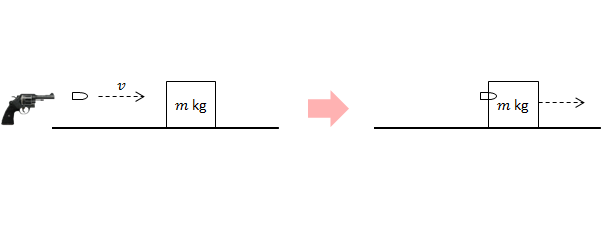Classical Mechanics

# Kinetic energy lost in inelastic collisions

An $\SI{8e3}{\kilo\gram}$ truck traveling at $20\text{ m/s}$ hits a parked car of mass $\SI{2e3}{\kilo\gram}$ from behind. If the collision is perfectly inelastic, what is the approximate total loss in kinetic energy?

Assume that the road is frictionless and ignore the deformation of the vehicles.A wooden block of mass $4.8\text{ kg}$ is at rest on a frictionless floor. A bullet of mass $0.2\text{ kg}$ is fired at $500\text{ m/s}$ towards the block. If the bullet becomes embedded inside the block, how much kinetic energy is lost?

A $150\text{ kg}$ bumper car travels at $12\text{ m/s}$ due east. It hits an identical bumper car traveling due north at $16\text{ m/s}.$ If the cars lock bumpers and stick together, how much kinetic energy is lost in total?

Two balls of masses $2\text{ kg}$ and $3\text{ kg},$ respectively, move in opposite directions on a frictionless floor. Their respective speeds are $2\text{ m/s}$ and $8\text{ m/s}.$ If they stick together after a collision, how much kinetic energy is lost in total?

An eagle of mass $18\text{ kg}$ is chasing a sparrow of mass $2\text{ kg}$ from straight behind with a constant speed of $13\text{ m/s}.$ The sparrow flies away at a constant speed of $7\text{ m/s}.$ A few seconds later, the sparrow is swallowed by the eagle. If air resistance is negligible, what is the total loss in kinetic energy?

×